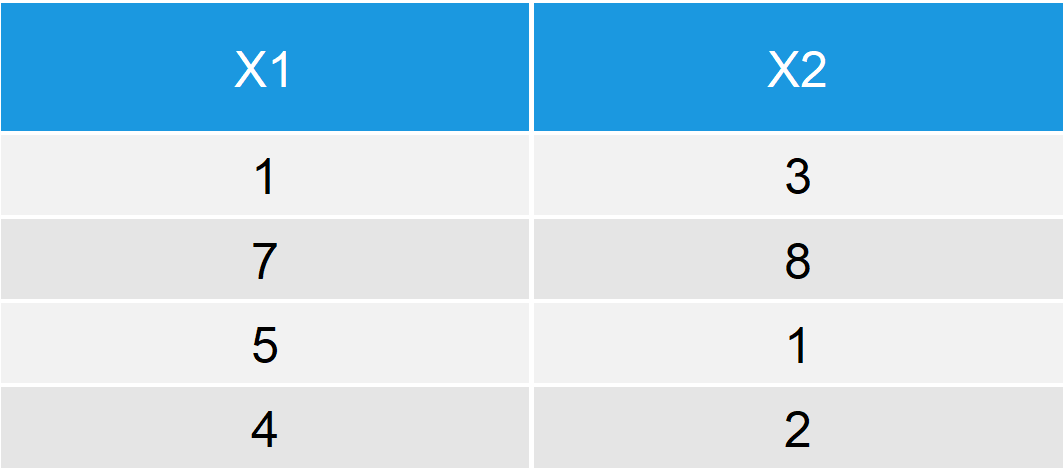# R transform Function (2 Example Codes) | Transformation of Data Frames

In this tutorial, I’ll explain you how to modify data with the transform function. Let’s first have a look at the basic R syntax and the definition of the function:

Basic R Syntax:

`transform(data, x = x_transformed)`

Definition:

The transform function modifies data.frames in a quick and easy way.

In the following, I’ll show in two examples how to apply the transform function in R. So without further explanations, let’s move on to the examples…

## Example 1: Use transform() to Convert Variables

The transform R function can be used to convert already existing variables of a data frame. Let’s first create an example data frame that we can use in the following examples:

```data <- data.frame(x1 = c(1, 7, 5, 4),            # Create example data frame
x2 = c(3, 8, 1, 2))
data                                              # Print data to RStudio console```Table 1: Example Data Frame.

Our data contains of two columns (numeric variables) and four rows. Now let’s use the transform function in order to convert the variable x1:

```data_ex1 <- transform(data, x1 = x1 + 10)         # Apply transform function
data_ex1                                          # Print data to RStudio console```Table 2: Convert Column of Data Frame.

As you can see in Table 2, we have added the value 10 to each of the elements of variable x1. An easy way to modify variables of data.frames!

Check out the following video, in case you need more explanations on the R code of Example 1. I’m explaining this example in the video:

Please accept YouTube cookies to play this video. By accepting you will be accessing content from YouTube, a service provided by an external third party.If you accept this notice, your choice will be saved and the page will refresh.

## Example 2: Add New Variable to Data

We can also use the transform command to concatenate a completely new variable to our data matrix. Have a look at the following R code:

```data_ex2 <- transform(data, x3 = c(5, 3, 3, 1))   # Apply transform function
data_ex2                                          # Print data to RStudio console```Table 3: Add Column to Data Frame.

As you can see, we have added a third column to our data.

## Video: Alternatives to the transform Function

In this R tutorial, I have shown you two ways of using transform in order to modify data.frames. However, the R programming language provides many different functions for data manipulation and depending on your specific needs other functions might be preferable. In fact, the transform function is much less popular than other functions such as cbind or rbind.

If you want to learn more about the transformation of data.frames, I can recommend the following video of the DataCamp YouTube channel. Have fun with the video and let me know in the comments, in case you have any questions about data manipulation in R.

Please accept YouTube cookies to play this video. By accepting you will be accessing content from YouTube, a service provided by an external third party.If you accept this notice, your choice will be saved and the page will refresh.

Subscribe to the Statistics Globe Newsletter

•Nara
April 6, 2019 1:41 am

Hi Joachim,
You are tutorials are excellent, short and crisp. Looking forward to seeing more on 1) data cleaning, 2) join functions, as well as 3) summary statistics when you have factor variables in the data frame.
Many thanks, Nara

•Nara
April 6, 2019 2:58 am

is it possible to have more demos on ‘tableone’ and ‘strata’ functions in r to create table and statistical summaries

•April 6, 2019 11:55 am

Thanks for the follow up comment. Your requests are noted on my to-do-list!

•February 8, 2022 9:26 pm

Hi ,I need to know how to generate data from half normal on R
i make transformation from normal to half normal but what can i do the next step ? please

•February 9, 2022 10:08 am